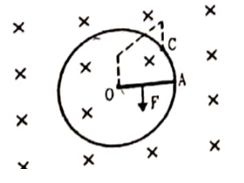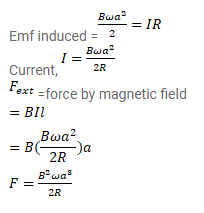# Figure shows a conducting circular loop of radius a placed in a uniform,

Question:

Figure shows a conducting circular loop of radius a placed in a uniform, perpendicular magnetic field B. A thick metal rod OA is pivoted at the center 0 . The other end of the rod touches the loop at A. The center $O$ and a fixed point $C$ on the loop are connected by a wire OC of resistance R. A force is applied at the middle of the rod OA perpendicularly, so that the rod rotates clockwise at a uniform angular velocity $\omega$. Find the force.Solution: